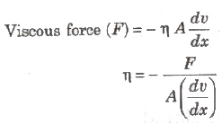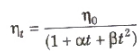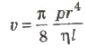Courses

# Viscosity and Poiseuille’s Formula Class 11 Notes | EduRev

## JEE : Viscosity and Poiseuille’s Formula Class 11 Notes | EduRev

The document Viscosity and Poiseuille’s Formula Class 11 Notes | EduRev is a part of the JEE Course Physics Class 11.
All you need of JEE at this link: JEE

Viscosity

The property of a fluid by virtue of which an internal frictional force acts between its different layers which opposes their relative motion is called viscosity.

These internal frictional force is called viscous force.

Viscous forces are inter-molecular forces acting between the molecules of different layers of liquid moving with different velocities.where, (dv/dx) = rate of change of velocity with distance called velocity gradient, A = area of cross-section and = coefficient of viscosity.

SI unit of η is Nsm-2 or pascal-second or decapoise. Its dimensional formula is [ML-1T-1].

The knowledge of the coefficient of viscosity of different oils and its variation with temperature helps us to select a suitable lubricant for a given machine.

Viscosity is due to transport of momentum. The value of viscosity (and compressibility) for ideal liquid is zero.

The viscosity of air and of some liquids are utilised for damping the given parts of some instruments.

The knowledge of viscosity of some organic liquids is used in determining the molecular weight and shape of large organic moleculars like proteins and cellulose.

Variation of Viscosity

The viscosity of liquids decreases with increase in temperaturewhere, η0 and ηt is are coefficient of viscosities at 0°C and t°C, α and β are constants.

The viscosity of gases increases with increase in temperatures as

η ∝ √T

The viscosity of liquids increases with increase in pressure but the viscosity of water decreases with increase in pressure.

The viscosity of gases do not changes with pressure.

Poiseuille’s Formula

The rate of flow (v) of liquid through a horizontal pipe for steady flow is given bywhere, p = pressure difference across the two ends of the tube. r = radius of the tube, n = coefficient of viscosity and 1 = length of the tube.

The Rate of Flow of Liquid

Rate of flow of liquid through a tube is given by

v = (P/R)

where, R = (8 ηl/πr4), called liquid resistance and p = liquid pressure.

Offer running on EduRev: Apply code STAYHOME200 to get INR 200 off on our premium plan EduRev Infinity!

## Physics Class 11

75 videos|312 docs|152 tests

,

,

,

,

,

,

,

,

,

,

,

,

,

,

,

,

,

,

,

,

,

;